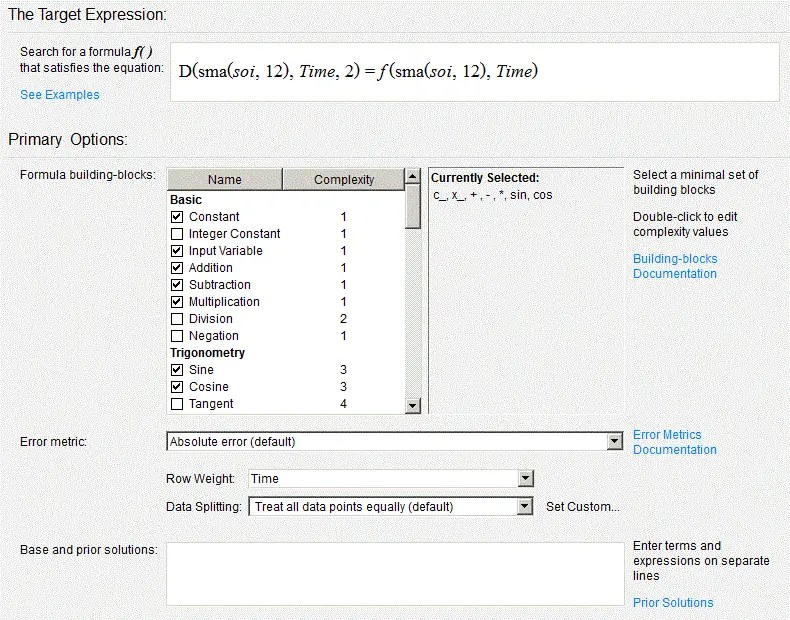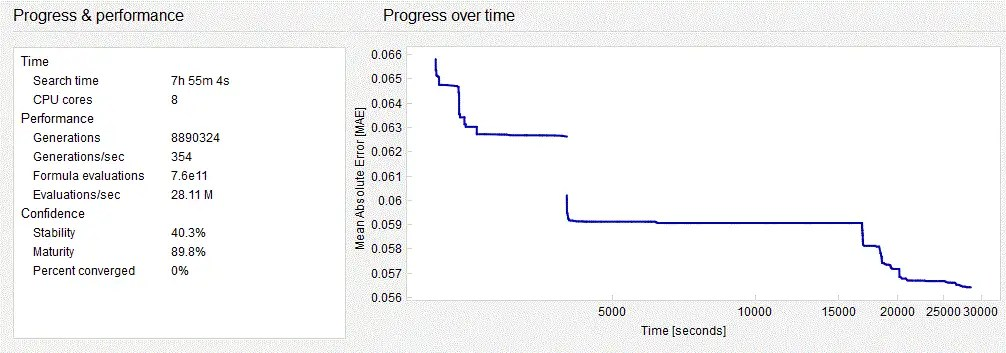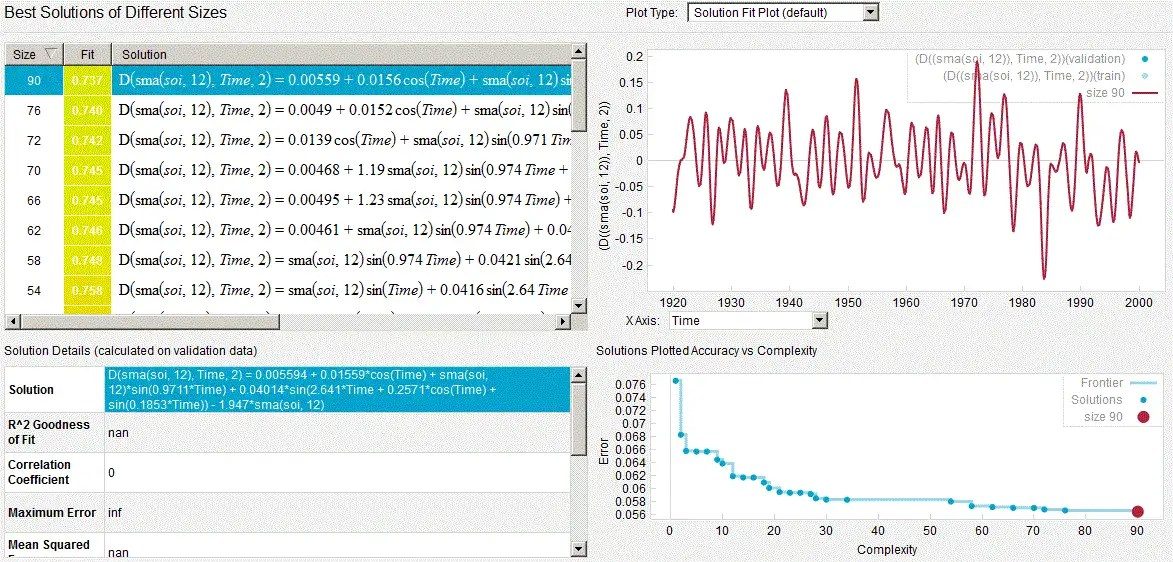# Eureqa!

Whenever I do machine learning (ML) experiments, I save the results for posterity. If I can’t make sense of them at the time, I will revisit later.

The following is a set of results from a Eureqa symbolic regression ML experiment on ENSO data from May of last year. The surprise is that it shouldn’t be a surprise, especially based on the fascinating Quasi-Biennial Oscillation (QBO) results of the last few months.

This is a standard experiment where I took official Southern Oscillation Index (SOI) measurements for a 100 year span from 1900 to 2000 and fed them into Eureqa. I used the target expression shown in Figure 1.   This is essentially the Eureqa recipe for finding a possible solution to the second order differential equation, i.e. the wave equation:$frac{{d^2 f(t)}}{{d t^2 }} + omega^2 f(t) = F(t)$Fig. 1: Eureqa target expression for SOI data. The SOI data is smoothed with a 12 month averaging routine (sma) to make the second derivative less noisy.

I let it run for about 8 hours as shown in Figure 2.  What it essentially performs is an evolutionary directed search over all possible forcing functions F(t) and wave equation coefficients applied to f(t).  Some say this is brute force, others say this is science and the way of the future .Fig. 2: Progress on solution

Figure 3 reveals the not very surprising surprise.  The highest complexity solution just happens to have a principle forcing function that aligns with the primary forcing on the QBO, and that happens to also be the (seasonally aliased) Draconic lunar month period of 27.212 days.Fig. 3:  Highest complexity solution found by Eureqa highlighted in blue on the left and the dot on the Pareto curve to the right. A correlation coefficient isn’t given because f(t) is used in fitting f”(t) (i.e. an implicit correlation).

This is the expanded result, with the (obviously) over-precise numbers as supplied by Eureqa:

```D(sma(soi, 12), Time, 2) = 0.00559435958083016 + 0.0155947948832677*cos(Time) + sma(soi, 12)*sin(0.971070066682118*Time) + 0.0401373411508968*sin(2.64123447957547*Time + 0.257149744031725*cos(Time) + sin(0.18533447928114*Time)) - 1.94747759165214*sma(soi, 12) ```

In the solution, there is a characteristic period of 4.5 years, and a Mathieu modulation of 6.47 years, the latter very close to the Chandler wobble period and the aliased Mf’ fortnightly tidal period of 13.606 days (half the Draconic period, giving 6.41 years when seasonally aliased).

The closeness of the main factor to the Draconic period is striking, much like that discovered for the QBO.

 2.64123 rads/yr aliased 27.2155 days Draconic 27.2122 days error=0.012%

Yet, the aliased Draconic (aka nodical) term has an additional frequency modulation, which causes the effective frequency to change over time from approximately 2.22 rads/yr to 3.05 rads/yr. See Figure 4 below, which is simply the derivative of the modulated sinusoid. The dashed and dotted lines correspond to unaliased Draconic months of 27.352 days and 27.084 days respectively.Fig. 4:  Taking the derivative of the modulated aliased Draconic cycle shows that the frequency  varies from 2.2 rads/year to 3.05 rads/yr.

Note that the variation in the Draconic cycle is well known and has a similar extent, 27.384 days (vs 27.352 days above) and 27.051 days (vs 27.084 days above). Very comparable effects!

http://eclipse.gsfc.nasa.gov/SEhelp/moonorbit.html

“The mean length of this nodical period is 27.21222 days (27d 05h 05m 36s). However, the actual duration can vary by over 6 h from the mean. Figure 4-9 plots the duration of the draconic month minus its mean value for 2008 through 2010. The shortest month over this 3-year period is 27.05115 days (27d 01h 14m), while the longest month is 27.38409 days (27d 09h 13m).”

There is also a potential strength modulation in the waveform due to the interaction with the tropical month (producing an 18.6 year beat period). Similarly for the anomalous month, which is more clearly seen in the QBO results.

One has to consider that the machine learning in this case is arguably free of bias, in the sense that a human is not forcing the outcome in any particular direction. The fact that the Eureqa data mining “robot” finds as a best fit this particular model, in what amounts to an aliased Draconic sinusoid with a slight modulation, is quite the parsimonious occurrence. What else to make of this but that the sloshing characteristics of ENSO are actually forced by the seasonally aliased rhythm of the lunar gravitational pull.

This all makes sense if both the QBO and ENSO have a common forcing, and the fact that in both cases, Eureqa found the same leading periodic term of 27.21 days when the cycles are unaliased from their seasonal reinforcement. Nature is really playing games with us if this is just a coincidence.

Yelling Eureqa! just about describes the situation.

## Notes

 After working with the CompSci department at the U of MN and witnessing how they work on climate science problems considering the vast amount of satellite data available, I have obviously become a believer in machine learning and data mining methods

## 3 thoughts on “Eureqa!”

1. Pingback: Scaling El Nino | context/Earth

2. Pingback: Daily Double | context/Earth